C Program to Calculate Wage of Labor on Daily Basis

In this tutorial you will learn about the C Program to Calculate Wage of Labor on Daily Basis and its application with practical example.

C Program to Calculate Wage of Labor on Daily Basis

In this tutorial, we will learn to create a C program that will Calculate the Wage of Labor on a Daily Basis using C programming.

Prerequisites

Before starting with this tutorial we assume that you are best aware of the following C programming topics:

• Operators in C Programming.
• Basic Input and Output function in C Programming.
• Basic C programming.
• While loop in C programming.
• Arithmetic operations in C Programming.

Program to Calculate Wage of Labor on Daily Basis

In c programming, it is possible to take numerical input from the user and Calculate the Wage of Labor on Daily Basis with the help of a very small amount of code. The C language has many types of header libraries which has supported function in them with the help of these files the programming is easy. But today we will Calculate the Wage of Labor on Daily Basis using c programming.

As per the following rule:

With the help of this program, we can Calculate the Wage of Labor on a Daily Basis.

Output:-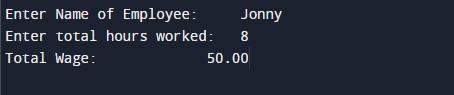In the above program, we have first initialized the required variable.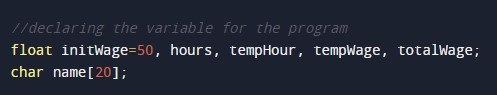• name = it will hold the character value for the name.
• initWage = it will hold the floating value for the workers.
• hours = it will hold the floating value for the working hours.
• temphour = it will hold the floating value.
• tempWage = it will hold the floating value.
• totalWage = it will hold the floating value to calculate the wages.

Input name and hour from the user.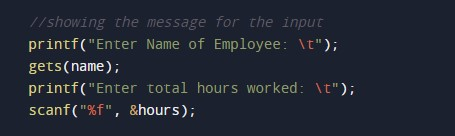Program Logic Code.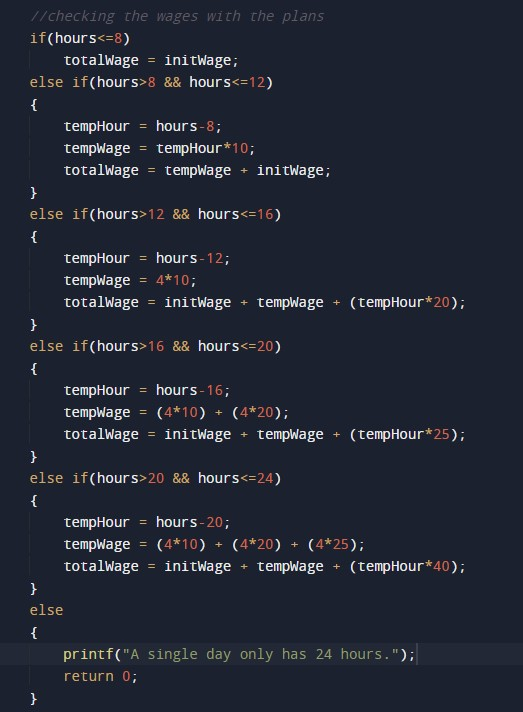Printing output for wages calculated.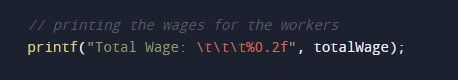In this tutorial we have learn about the C Program to Calculate Wage of Labor on Daily Basis and its application with practical example. I hope you will like this tutorial.